# Geometry Help: Problem 29

 Before beginning the solution of Problem 29, we need to be aware of the following preliminary propositions: DEFINITION 1. Midpoint is the point on a line segment dividing it into two segments of equal length.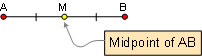DEFINITION 2. Angle Bisector is a ray that divides the angle into two congruent adjacent angles.DEFINITION 3. Triangle is a three side polygon. Polygon is a closed plane figure with n sides. Altitude is the perpendicular line segment from one vertex to the line that contains the opposite side.PROPOSITION 1. If two lines are parallel, each pair of alternate interior angles are congruent. Also converse.PROPOSITION 2. Two acute angles are congruent if their sides are respectively perpendicular to each other.PROPOSITION 3. The sum of the measures of the three angles of a triangle is 180.PROPOSITION 4. The measure of an exterior angle of a triangle equals the sum of the measures of the two non-adjacent interior angles.PROPOSITION 5. The sum of the measures of the acute angles of a right triangle is 90 (complementary).PROPOSITION 6. Triangle Congruence S.A.S. If two sides and the included angle of one triangle are congruent to the corresponding parts of another, then the triangles are congruent.PROPOSITION 7. Triangle Congruence A.S.A. If two angles and the included side of one triangle are congruent to the corresponding parts of another, then the triangles are congruent.PROPOSITION 8. Triangle Congruence S.S.S. If three sides of one triangle are congruent to the three sides of a second triangle, then the triangles are congruent.PROPOSITION 9. Any point on the bisector of an angle is equidistant from the sides of the angle.PROPOSITION 10. If a line is tangent to a circle, it is perpendicular to a radius at the point of tangency.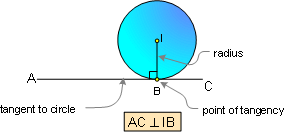PROPOSITION 11. The bisectors AD, BF and CE of the angles of a triangle ABC meet in a point I, which is equidistant from the sides of the triangle. The incircle is the inscribed circle of a triangle. The center of the incircle is called the incenter, and the radius of the circle is called the inradius.PROPOSITION 12. The circumcenter O of the triangle ABC is the concurrence point of the three perpendicular bisectors of the sides. The circumcenter has the same distance to the three vertices.PROPOSITION 13. In the same or congruent circles, congruent arcs have congruent chords. Also converse.PROPOSITION 14. Two tangent segments to a circle from an external point are congruent.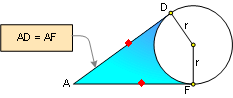PROPOSITION 15. Isosceles triangle: If two sides of a triangle are congruent, the angles opposite these sides are congruent. Also converse.PROPOSITION 16. For a right triangle with catheti (legs) a and b, the hypotenuse c, and the inradius r: a + b = c + 2r.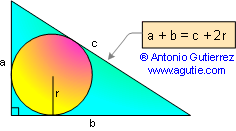PROPOSITION 17. Right triangle 45-45-90: the measure of either leg equals one-half the measure of the hypotenuse time.PROPOSITION 18. A central angle is measured by its intercepted arc.PROPOSITION 19. An inscribed angle is measured by one-half its intercepted arc.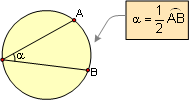PROPOSITION 20. A cyclic quadrilateral is a quadrilateral whose vertices all lie on a single circle. Opposite angles of a cyclic (inscribed) quadrilateral are supplementary. Also converse.Home | Geometry | Search | Problems | Email | By Antonio Gutierrez Last updated: June 27, 2009